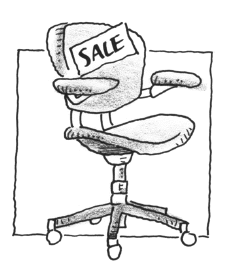### Home > CALC > Chapter 12 > Lesson 12.2.1 > Problem12-73

12-73.

Acme office furniture is selling computer chairs for $145$ each, and they are looking for ways to increase sales revenue. They have found that companies have bought an average of $64$ chairs per order, so they decide to offer a lower price on larger orders. They are considering the following plan: the cost will be $145$ per chair for $80$ or fewer chairs, but for each chair over $80$, they will give a discount of $50¢$ per chair on the entire order. What is the maximum amount that any customer would have to pay for an order of chairs under this plan?1. $17{,}112.50$

1. $13{,}912.50$

1. $21{,}312.50$

1. $145.00$

1. There is no maximum amount.

Only consider orders $≥ 80$ chairs.
The cost of the chairs before the discount is $145x$ for $x$ chairs.
The discount for $81$ chairs is $0.50(1)(81)$.
The discount on $82$ chairs is $0.50(2)(82)$.
The discount on $83$ chairs is $0.50(3)(83)$.
...
The discount amount is $0.50(x-80)x$.

Write an equation for the total cost, including the discount.
Then maximize the equation.
Remember, $x =$ number of chairs and the problem asks for the cost.